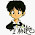## Saturday, November 15, 2014

### [LeetCode] Container With Most Water

Given n non-negative integers a1a2, ..., an, where each represents a point at coordinate (iai). n vertical lines are drawn such that the two endpoints of line i is at (iai) and (i, 0). Find two lines, which together with x-axis forms a container, such that the container contains the most water.
Note: You may not slant the container.

(1) min(a(left),aj) <= a(left) = min(a(left), a(right))
(2) j-left < right-left

left = 0, right = n-1
(1) a[left] < a[right], left++
(2) a[left] > a[right], right--
(3) a[left] = a[right], left++, right--

 ``` 1 2 3 4 5 6 7 8 9 10 11 12 13 14 15 16 17 18 19 20``` ```class Solution { public: int maxArea(vector &height) { int mxArea = 0; int left = 0, right = height.size()-1; while(leftheight[right]) right--; else { left++; right--; } } return mxArea; } }; ```

1.2.3.